# Magnetic Moment

Also found in: Dictionary, Thesaurus, Medical, Wikipedia.

## magnetic moment

[mag′ned·ik ′mō·mənt]
(electromagnetism)
McGraw-Hill Dictionary of Scientific & Technical Terms, 6E, Copyright © 2003 by The McGraw-Hill Companies, Inc.
The following article is from The Great Soviet Encyclopedia (1979). It might be outdated or ideologically biased.

## Magnetic Moment

a fundamental quantity characterizing the magnetic properties of matter.

According to the classical theory of electromagnetic phenomena, electric macrocurrents and microcurrents are the source of magnetism, and a closed circuit is considered the elementary source. It follows from experiments and the classical theory of electromagnetic fields that the magnetic effects of a closed circuit (one carrying a current) are defined if the product (M) of the current strength i and the area of the circuit σ is known (M = iσ /c in the cgs system, where c is the speed of light); the vector M is by definition the magnetic moment. The relationship may also be written in a different form: M = ml, where m is the equivalent magnetic charge of the circuit and 1 is the distance between charges of opposite sign (+ and —).

Elementary particles, atomic nuclei, and the electron shells of atoms and molecules all have magnetic moments. As quantum mechanics has shown, the magnetic moment of elementary particles (electrons, protons, neutrons) is determined by the existence in them of an intrinsic mechanical moment, or spin. The magnetic moment of a nucleus is the sum of the intrinsic (spin) magnetic moments of the protons and neutrons that form the nucleus and of the magnetic moments associated with the orbital motion of the protons and neutrons within the nucleus. The magnetic moments of the electron shells of atoms and molecules consist of the spin and orbital magnetic moments of the electrons.

The spin magnetic moment of an electron μsp can have two equal and opposite projections onto the direction of an external magnetic field H. The absolute value of the projection is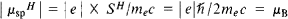where μB = (9.274096+0.000065) ×10-21 ergs per gauss, the Bohr magneton; h = h/2π, where h is Planck’s constant; e and me are the charge and mass of the electron, respectively; c is the speed of light; and SH is the projection of the spin mechanical moment onto the field direction H. The absolute value of the spin magnetic moment is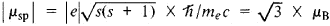where s = 1/2, the spin quantum number. The ratio of the spin magnetic moment to the mechanical moment (spin) is

gsp = ǀµspǀ/ǀSǀ = ǀeǀ/mec

since the spin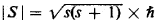Studies of atomic spectra have demonstrated that μspH is actually equal not to JULB but to μB (1 + 0.0116), owing to the effect of zero-point vibrations of the electromagnetic field on the electron.

The orbital magnetic moment of the electron μorb is related to the mechanical orbital moment Morb by the equation gorb = ǀμorbǀ/Morb ǀ = ǀeǀ/2mc; that is, the magnetomechanical ratio gorb is half the gsp. Quantum mechanics admits only a discrete number of possible projections of the μ,orb onto the direction of an external field (space quantization): μ,Horb = m1 ×μB1 where m1 is the magnetic quantum number, which assumes 2l + 1 values (0, ±1, ±2, … , ±l, where l is the orbital quantum number).

In many-electron atoms, the orbital and spin magnetic moments are determined by the quantum numbers L and S of the combined orbital and spin moments. The summation of these moments is carried out according to the rules of space quantization. Because of the inequality of the magnetomechanical ratios for the spin of an electron and its orbital motion (gsp ≠ grb), the net magnetic moment of the atomic shell will be neither parallel nor antiparallel to its net mechanical moment J. Therefore, it is often the component of the total magnetic moment in the direction of the vector J, equal to μj =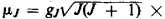×μB (where gj, is the magnetomechanical ratio of the electron shell and J is the total orbital quantum number), that is considered.

The magnetic moment of a proton, the spin of which is equal to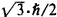should by analogy to the electron be equal to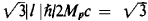μnuc (where Mp is the mass of the proton, which is 1836.5 times greater than me and μnuc is the nuclear magneton, equal to l/1836.5μμB). A neutron should have no magnetic moment, since it has no charge. Experiments have shown, however, that the magnetic moment of a proton μP = 2.7927μnuc and that the magnetic moment of a neutron μn = —1.91315μnuc, owing to the presence of meson fields near the nucleons, which determine the specific nuclear interactions of the neutrons and influence their electromagnetic properties. The total magnetic moments of complex atomic nuclei are not multiples of μnuc nor of μp and μn; for example, the magnetic moment of the nucleus of potassium 1940K equals — 1.29μnuc. The influence of the nuclear forces acting between the nucleons that make up the nucleus is the reason for this nonadditivity. The magnetic moment of the atom as a whole is equal to the vector sum of the magnetic moments of the electron shell and the atomic nucleus.

The average value of the net magnetic moment of all microparticles forming a body is calculated in order to characterize the magnetic state of a macroscopic body. The magnetic moment per unit volume of a body is called the magnetization. The concept of average atomic magnetic moment as the average value of the magnetic moment per atom (ion)—the carrier of the magnetic moment in the body—is introduced for macrobodies, especially in the case of those that exhibit atomic magnetic ordering (ferromagnetic, ferrimagnetic, and antiferromagnetic substances). In substances with magnetic ordering, these average atomic magnetic moments are obtained as the quotient of the spontaneous magnetization of the ferromagnetic bodies or magnetic sublattices in ferrimagnets and antiferromagnets (at a temperature of absolute zero) and the number of atoms—the carriers of the magnetic moment—per unit volume.

These average atomic magnetic moments usually differ from the magnetic moments of isolated atoms; in terms of Bohr magnetons JLLB, their values are fractional. (For example, in the transition d metals iron, cobalt, and nickel, they are, respectively, 2.218 μB, 1.175 μB and 0.604 μB.) This difference is due to a change in the motion of the d electrons (the carriers of the magnetic moment) in a crystal in comparison to their motion in isolated atoms. In the case of rare-earth metals (the lanthanides) and nonmetallic ferromagnetic or ferrimagnetic compounds (for example, the ferrites), the incomplete d orf layers of the electron shell (the primary atomic carriers of the magnetic moment) of neighboring ions in the crystal overlap slightly; therefore, there is no appreciable collectivization of the layers (as in d metals), and the magnetic moments of these bodies differ little in comparison to isolated atoms.

Direct experimental determination of the magnetic moment of the atoms in a crystal has become possible as a result of the methods of magnetic neutron diffraction and microwave spectroscopy (nuclear magnetic resonance, electron paramagnetic resonance, ferromagnetic resonance) and the application of the Mössbauer effect. For paramagnetic substances, the concept of average atomic magnetic moment, which is defined in terms of the experimentally determined Curie constant entering into the formula for Curie’s law (or the Curie-Weiss law), may also be used.

### REFERENCES

Tamm, I. E. Osnovy teorii elektrichestva, 8th ed. Moscow, 1966.
Landau, L. D., and E. M. Lifshits. Elektrodinamika sploshnykh sred. Moscow, 1959.
Dorfman, Ia. G. Magnitnyesvoistva istroenie veshchestva. Moscow, 1955.
Vonsovskii, S. V. Magnetizm mikrochastits. Moscow, 1973.

S. V. VONSOVSKII

The Great Soviet Encyclopedia, 3rd Edition (1970-1979). © 2010 The Gale Group, Inc. All rights reserved.
References in periodicals archive ?
Octahedral geometry was suggested for all complexes  by observing electronic spectra and magnetic moment values (Table 1), which was further strengthened by the low value of molar conductance, indicating the complexes had a non-electrolytic nature.
At b = 80 mm, this leads to relatively small magnetic moments [M.sub.z] = 0.5 / 2.8 A x [m.sup.2] (Fig.
However, in the calculation of magnetic moment, the rotational velocity decreases by a factor of g, while the equivalent radius increases by a factor approximately equal to g squared.
where [B.sub.a] is the total magnetic anomaly (vehicular target) due to magnetization, m is the vehicular magnetic moment, and r is a proximity distance between a vehicle and wireless sensor node.
In the adopted SDW approximation, we assume a zero contribution to the magnetization from atoms with the SDW-generating magnetic moments. With consideration for the fraction and the average HFF <[B.sub.hf] (F)) of the ferromagnetic phase, the magnetic moment [bar.m] is estimated to be 0.42 [[mu].sub.B]/Fe for [Fe.sub.65][Al.sub.35] and 0.8 [[mu].sub.B]/Fe for [Fe.sub.65][Al.sub.30][Ga.sub.5].
Then, the effective neutrino magnetic moment is written in mass basis through a proper rotation; for a detailed description of this formalism see .
High concentrations of defects were observed in all the samples, indicating the possible role of defects in inducing magnetic moments and mediating ferromagnetism.
To achieve this, we implement a set of equations which describe the magnetic field during the short disturbance, which occurs due to the moving magnetic moment. The dataset for the optimization is centered at the gradient peak and is usually 10 + 10 seconds long (Figure 4).
"Our experiment has shown, based on a measurement six times more precise than any done before, that the standard model holds up, and that there seems in fact to be no difference in the proton/antiproton magnetic moments at the achieved measurement uncertainty.
The ferromagnetism can be considered a special case of paramagnetism in which the magnetic moments of individual atoms align, and all point in the same direction.
The magnetic moment of 1.53 BM of the glycinato Cu(II) complex is indicative of an antiferromagnetic spin-spin interaction through molecular association with possible Cu-Cu interaction or dimerization; similar results have been reported for the copper(II)acetate complex [33, 62].

Site: Follow: Share:
Open / Close